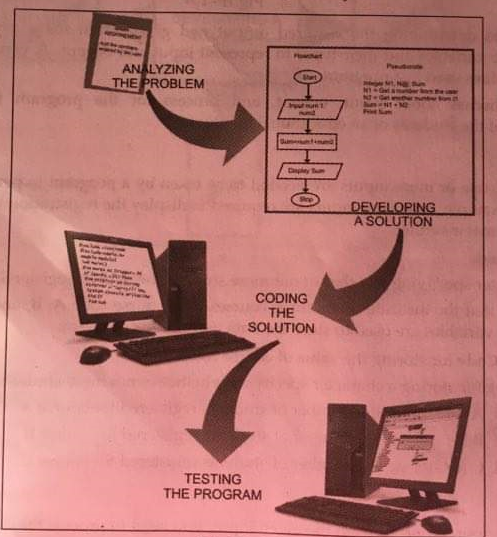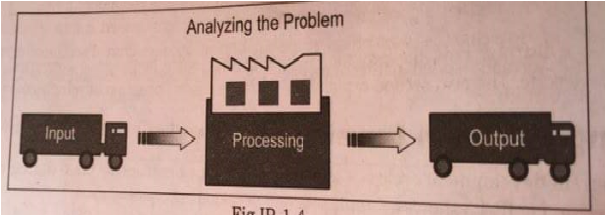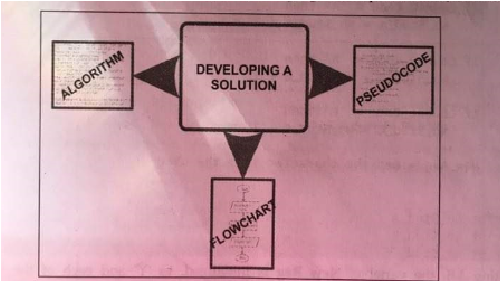# Software Engineering | Program Development Life Cycle

A program development cycle is a series of tasks or activities that take place during the development of a program. Fig.IP-1.3 shows a sample program development cycle.As shown in Fig.IP-1.3, the various stages of the program development cycle as follows:

1. Analyzing the problem
2. Developing a solution
3. Coding the solution
4. Testing the program

Now, let's discuss each of these stages of the program development cycle by considering a program that displays registration information of the students of an educational institute.

Analyzing the Problem

Analyzing the problem involves determining what the program need to perform and what you need to do to enable that program to perform that task. Once you have clearly visualized the problem, you can move on to design the solution for the program. While analyzing a problem, you need to consider:

The input supplied
A process to obtain the required output from the given input
The output desired

Fig.IP-1.4 shows how these three are related to each other:In addition to determining the required output and given input for a program, you also need to determine the variables and their types to represent input and output. A variable acts as a placeholder for storing values used in a program.

Let's now discuss about input, output, and process for the program that displays registration information of the students of an educational institute.

Input

As explained, one or more inputs are needed to be taken by a programs to perform a task and return an output. For example, following inputs are required to display the registration information of the students of an educational institute:

⦁  Course code
⦁  A character specifiying whether or not more students need to be registered

Let's assume that the institute offers three courses with course code A, B, and C. With the assumption, the following variables are used to store the data used in this program:

Course_Code for storing the value of course code
New_Reg  for storing a character specifying whether or not more students need to be registers
Not_Stud_A for storing the number of students registered for course A
No_Stud_B for storing the number of students registered for course B
No­_Stud_C for toring the number of students registered for course C

Process

To obtain the desired output from the given input, you need to process the data. The steps processing the data in this program are as follows:

1.  First, check the course code for the first student.
2.  If it is A, the entry will be made to the respective course, that is, course A. In this way, the course code for all the students is checked and the entries in the respective courses are made.
3.  Finally, the total number of entries for the three courses is displayed.

Output

The output of the program includes:
Total number of students registered for course A
Total number of students registered for course B
Total number of students registered for course C

Developing a Solution

Once the problem has been analyzed, that is, the required output and the given input are determined, you can start developing a solution for the problem. The most important task in developing a solution is the development of logic that solves the problem. This requires creation of a step-by-step procedure (or a set of instructions) to solve the problem. This type of procedure is commonly termed as an algorithm. Once you have created an algorithm to solve a problem, you can convert it into either a flowchart or pseudocode. A flowchart is a graphical representation of an algorithm. Pseudocode refers to short, readable, and formally-styled language code that is used to explain specific tasks within a program's algorithm.

Fig.IP-1.5 illustrates how a solution is developed by using algorithm, pseudocode, and flowchart:#### Sundar Neupane

I like working on projects with a team that cares about creating beautiful and usable interfaces.